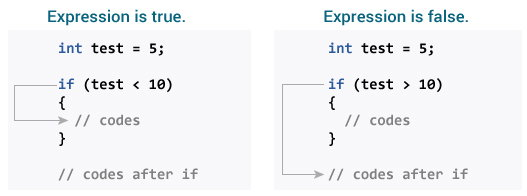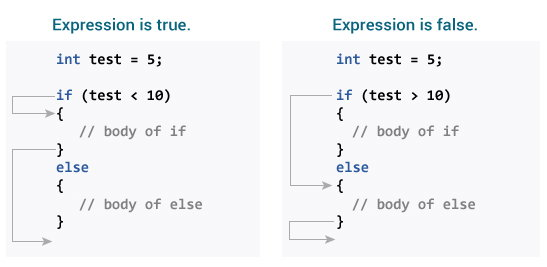# What is a if else statement in c

## C if Statement

The syntax of the `if` statement in C programming is:
```
`if (test expression) `
`{`
`   // statements to be executed if the test expression is true`
`}`

```

### How if statement works?

The `if` statement evaluates the test expression inside the parenthesis `()`.
• If the test expression is evaluated to true, statements inside the body of `if` are executed.
• If the test expression is evaluated to false, statements inside the body of `if` are not executed.To learn more about when test expression is evaluated to true (non-zero value) and false (0), check relational and logical operators.

### Example 1: if statement

```
`// Program to display a number if it is negative`

`#include <stdio.h>`
`int main() {`
`    int number;`

`    printf("Enter an integer: ");`
`    scanf("%d", &number);`

`    // true if number is less than 0`
`    if (number < 0) {`
`        printf("You entered %d.\n", number);`
`    }`

`    printf("The if statement is easy.");`

`    return 0;`
`}`

```
Output 1
```Enter an integer: -2
You entered -2.
The if statement is easy.```
When the user enters -2, the test expression `number<0` is evaluated to true. Hence, You entered -2 is displayed on the screen.
Output 2
```Enter an integer: 5
The if statement is easy.```
When the user enters 5, the test expression `number<0` is evaluated to false and the statement inside the body of `if` is not executed

## C if...else Statement

The `if` statement may have an optional `else` block. The syntax of the `if..else` statement is:
```
`if (test expression) {`
`    // statements to be executed if the test expression is true`
`}`
`else {`
`    // statements to be executed if the test expression is false`
`}`

```

### How if...else statement works?

If the test expression is evaluated to true,
• statements inside the body of `if` are executed.
• statements inside the body of `else` are skipped from execution.
If the test expression is evaluated to false,
• statements inside the body of `else` are executed
• statements inside the body of `if` are skipped from execution.### Example 2: if...else statement

```
`// Check whether an integer is odd or even`

`#include <stdio.h>`
`int main() {`
`    int number;`
`    printf("Enter an integer: ");`
`    scanf("%d", &number);`

`    // True if the remainder is 0`
`    if  (number%2 == 0) {`
`        printf("%d is an even integer.",number);`
`    }`
`    else {`
`        printf("%d is an odd integer.",number);`
`    }`

`    return 0;`
`}`

```